CBSE Class 12 Sample Paper for 2021 Boards

Class 12
Solutions of Sample Papers and Past Year Papers - for Class 12 Boards

## In an office three employees Vinay, Sonia and Iqbal process incoming copies of a certain form. Vinay process 50% of the forms. Sonia processes 20% and Iqbal the remaining 30% of the forms. Vinay has an error rate of 0.06, Sonia has an error rate of 0.04 and Iqbal has an error rate of 0.03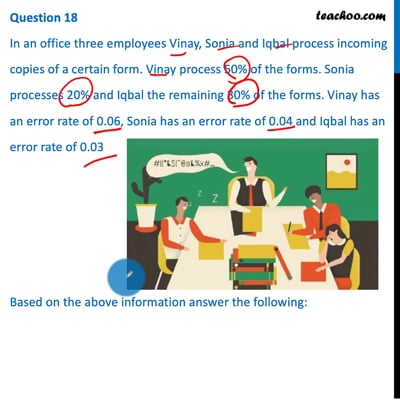This video is only available for Teachoo black users

## d) 0.06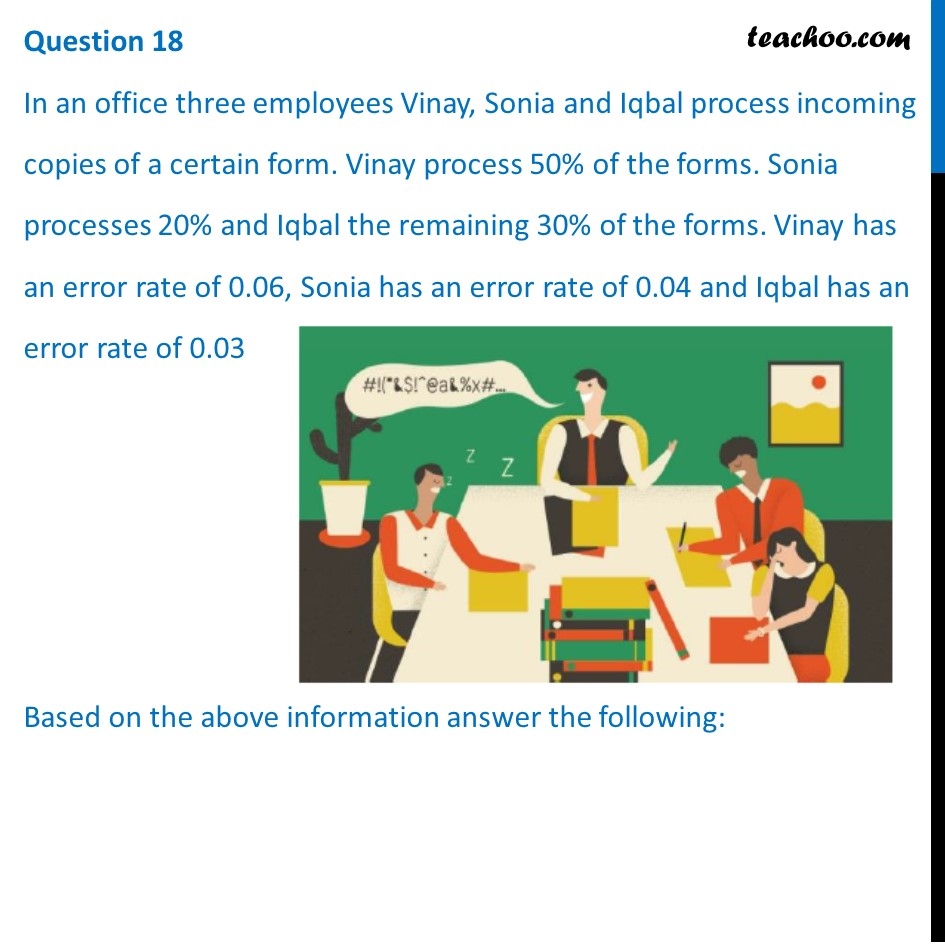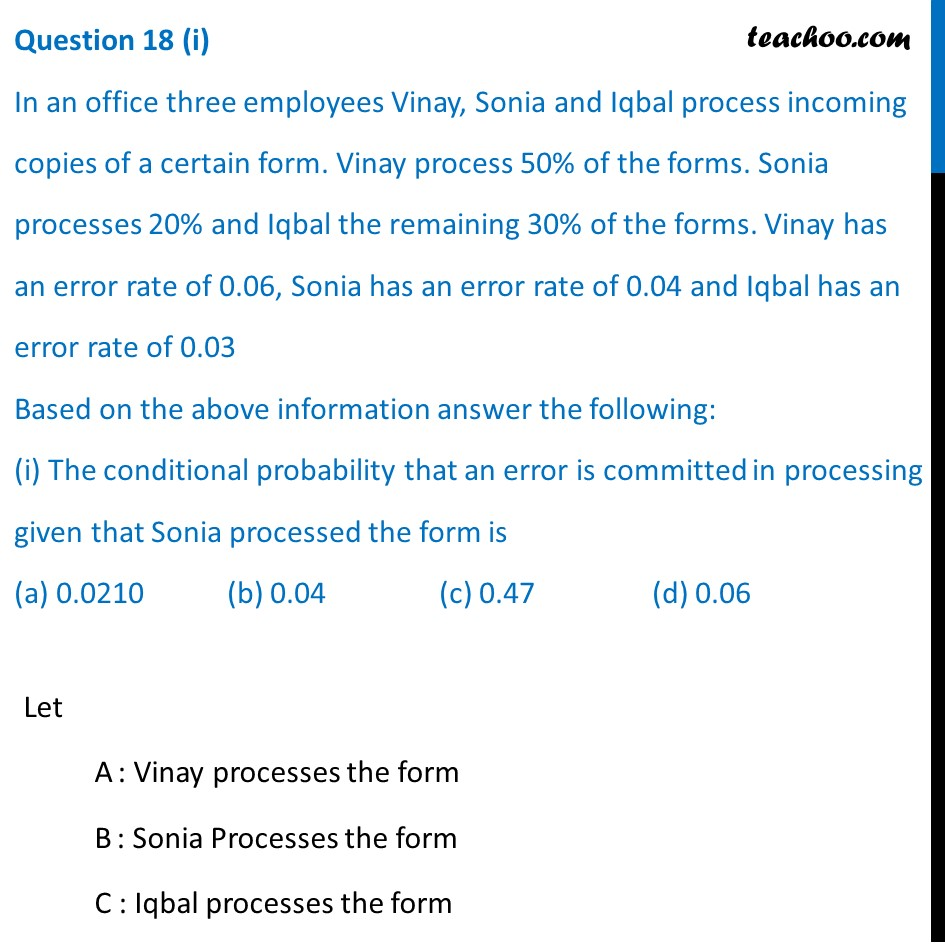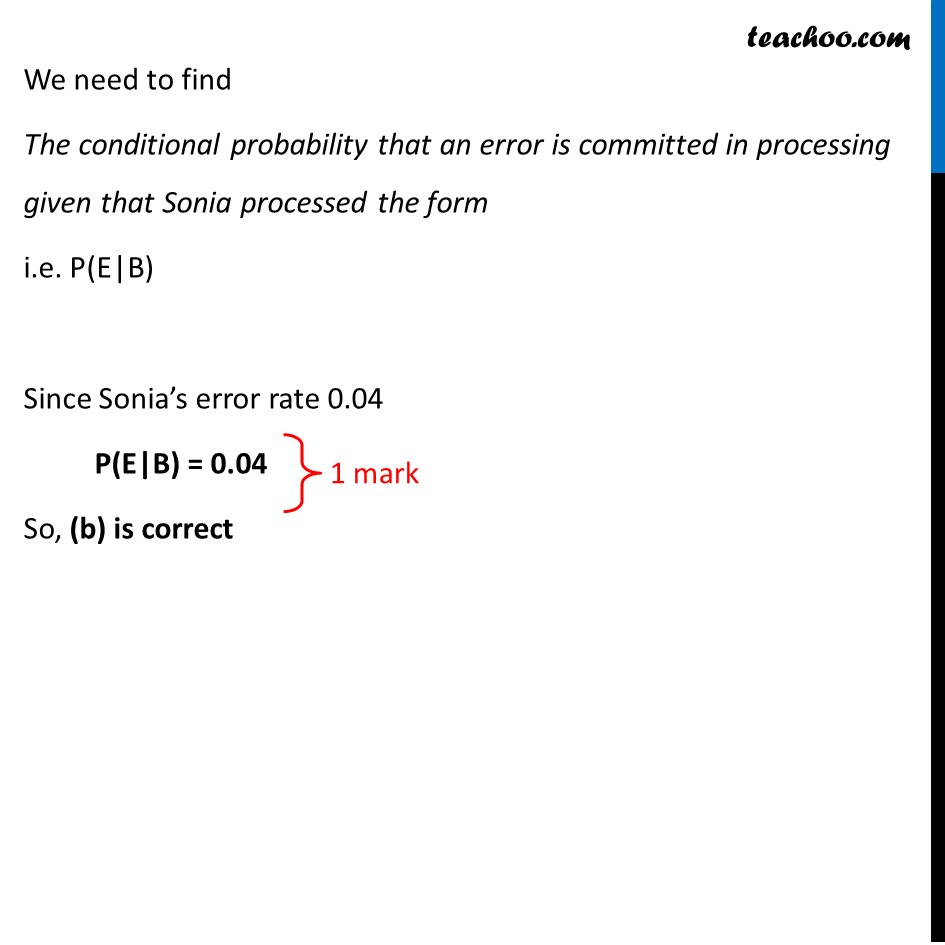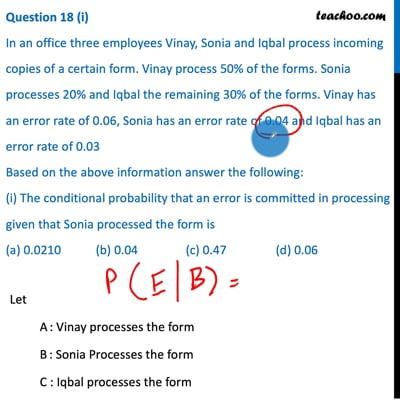This video is only available for Teachoo black users

## d) 0.68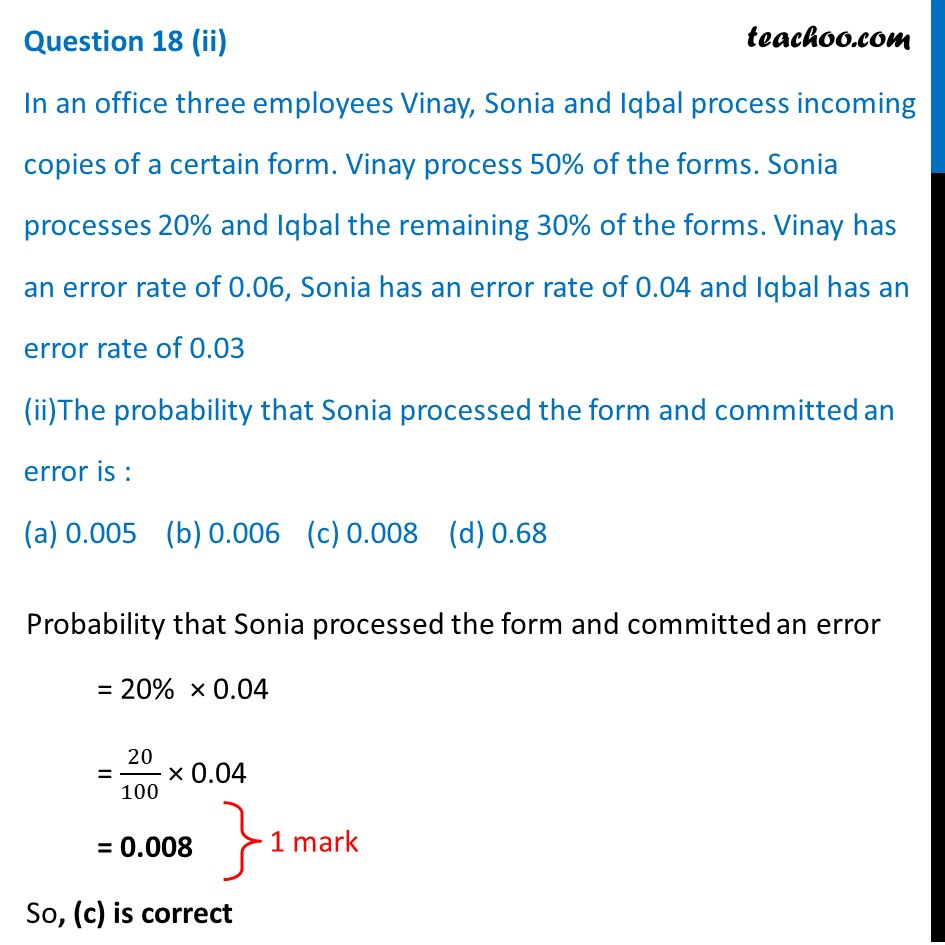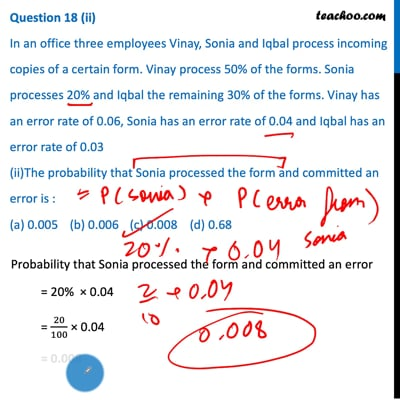This video is only available for Teachoo black users

## d) 1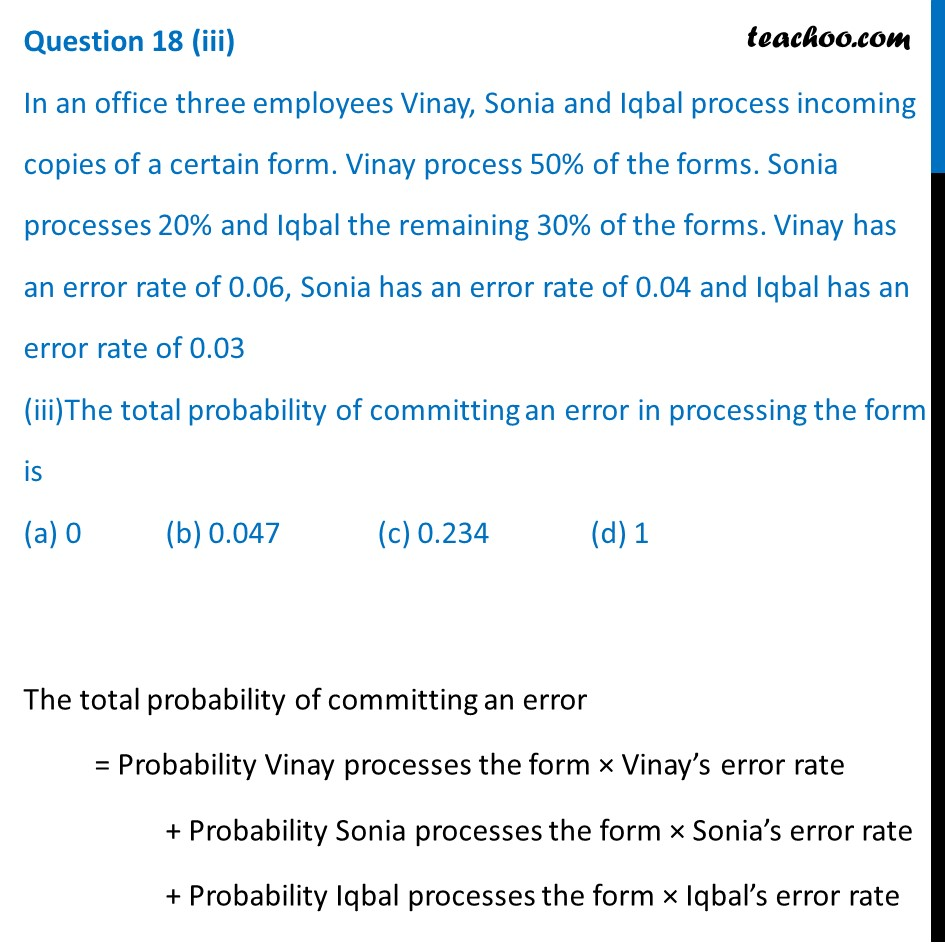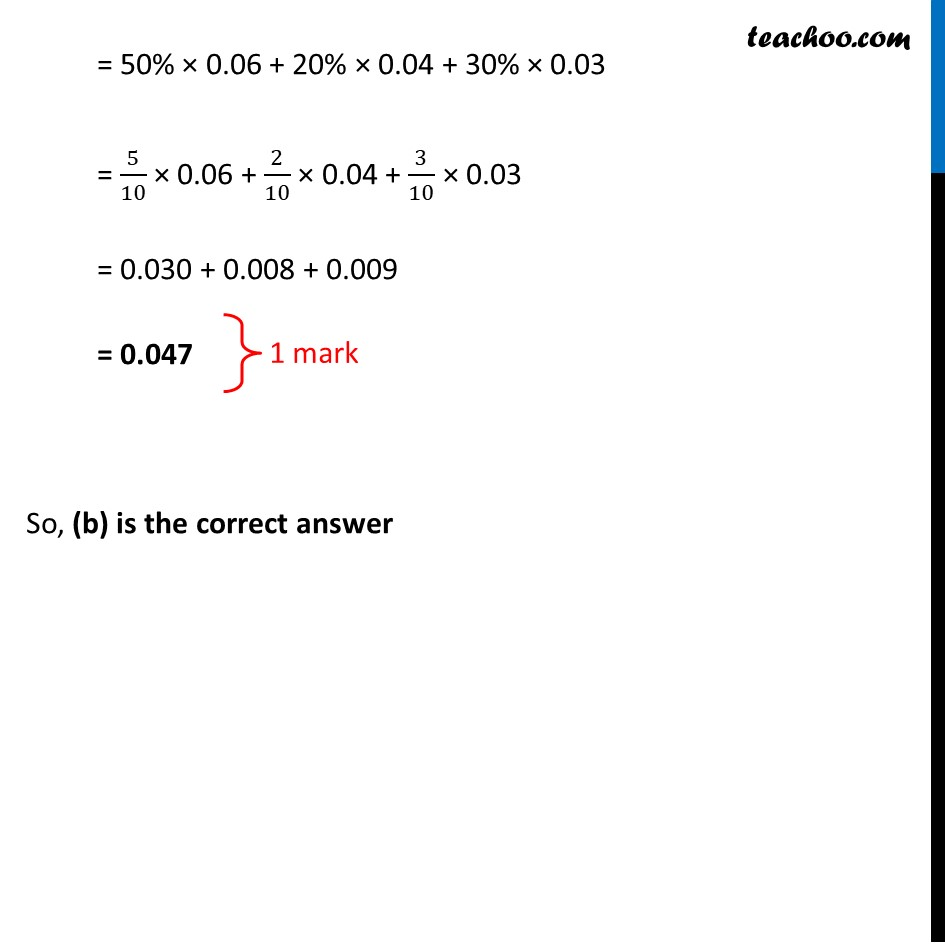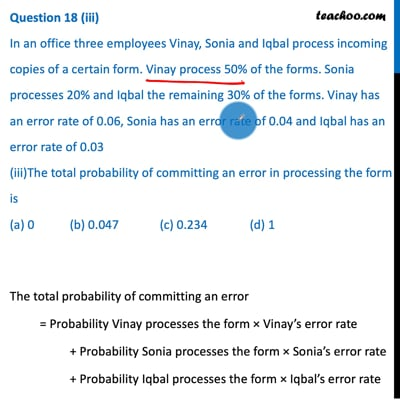This video is only available for Teachoo black users

## d) 17/47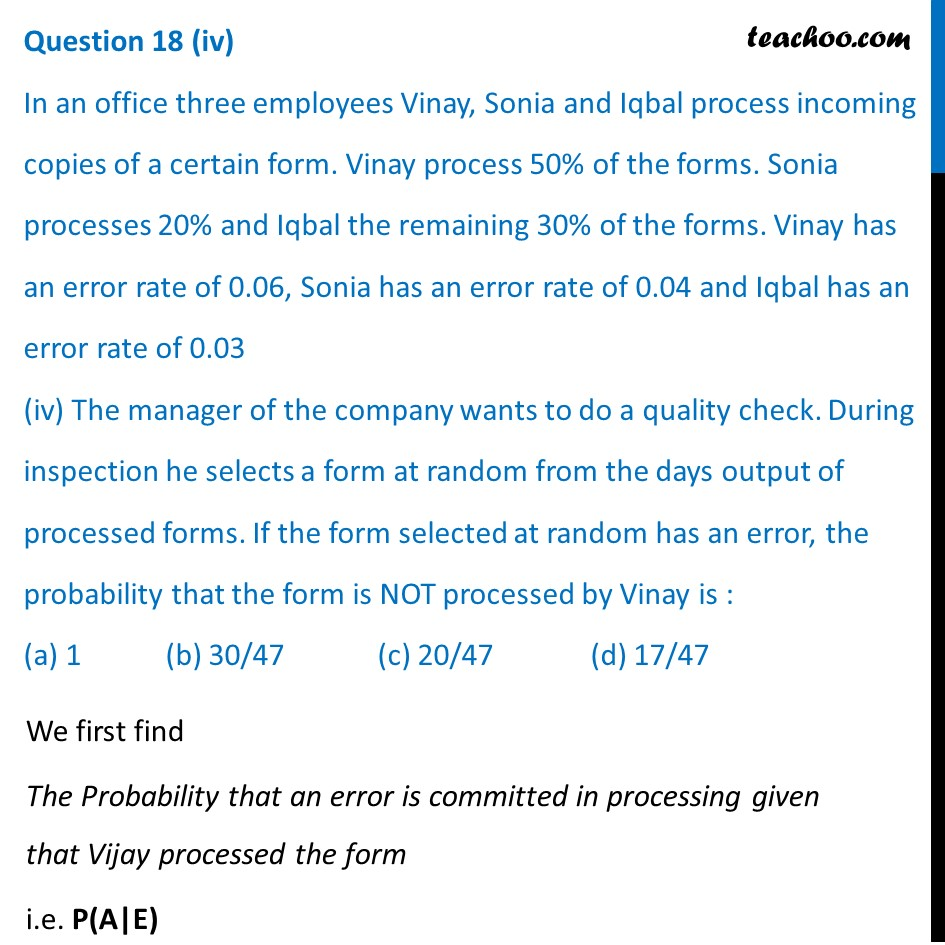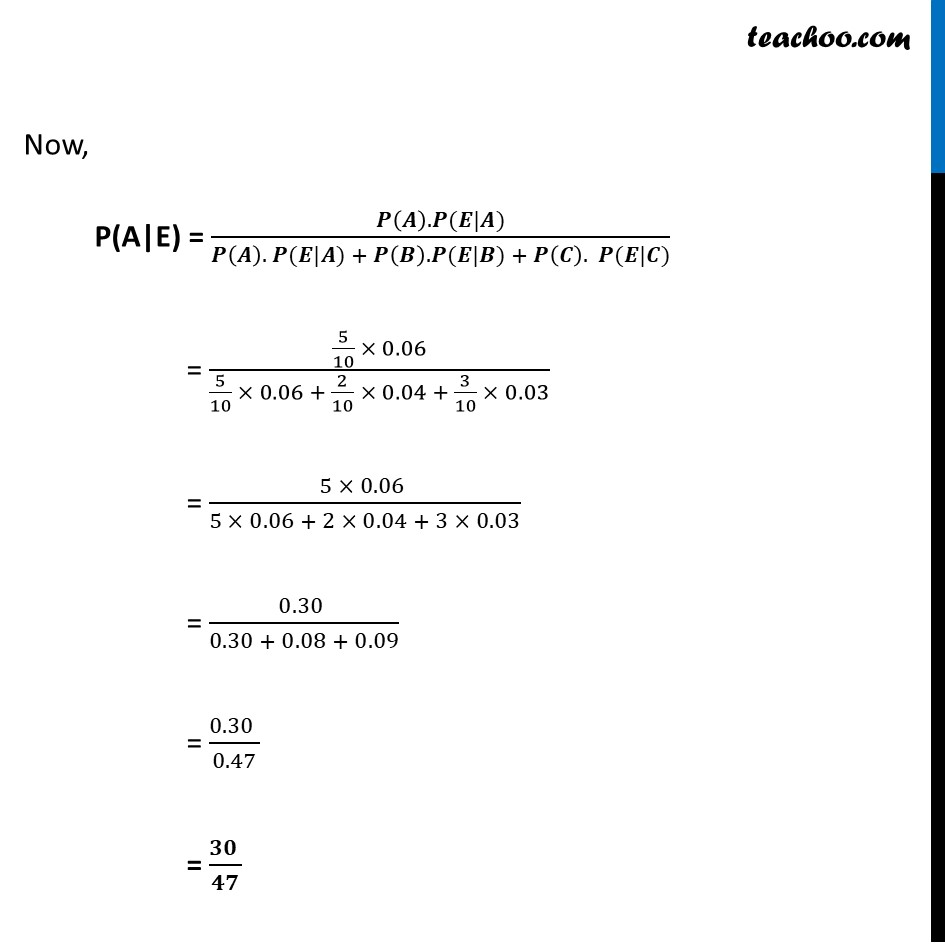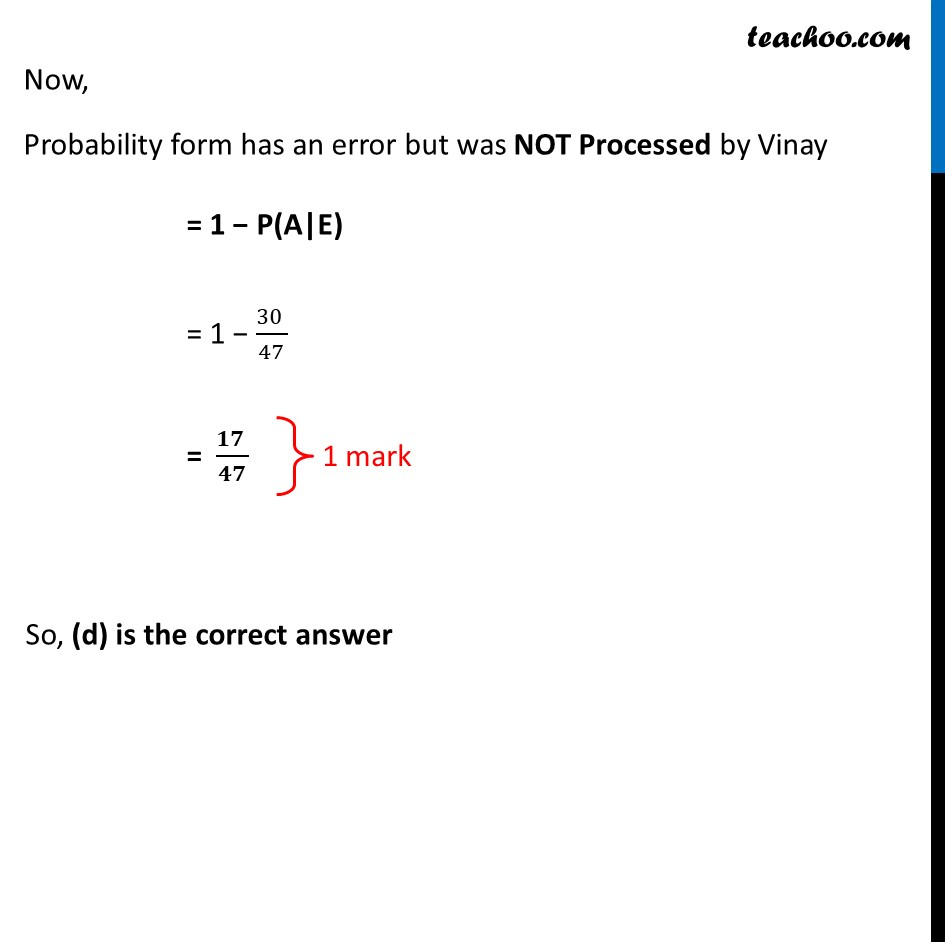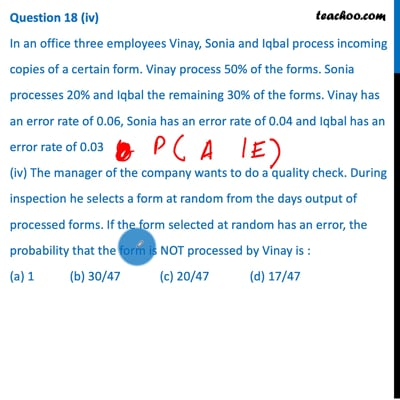This video is only available for Teachoo black users

## d) 1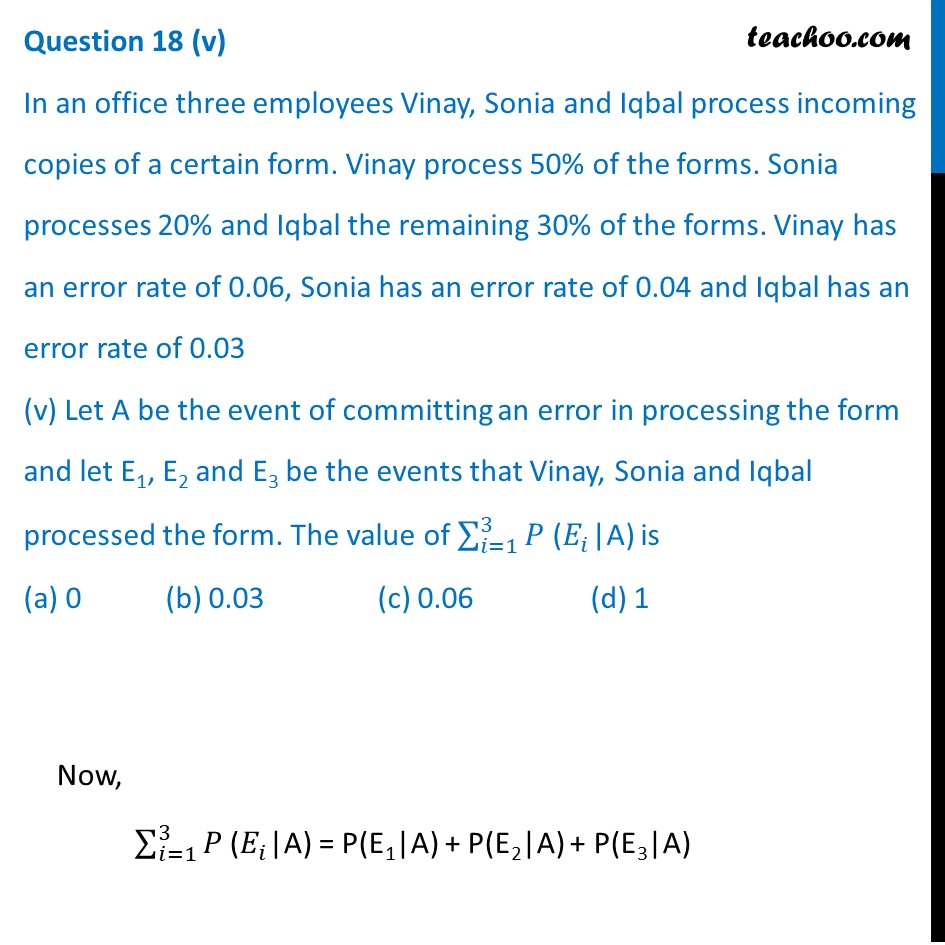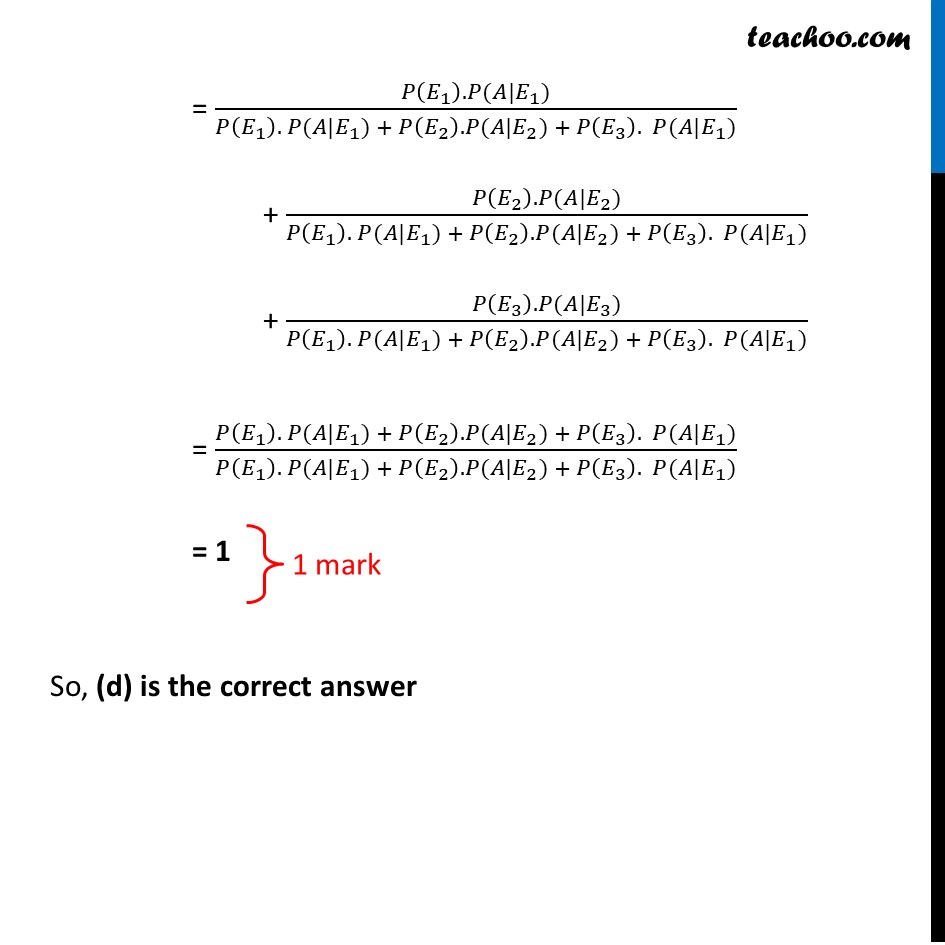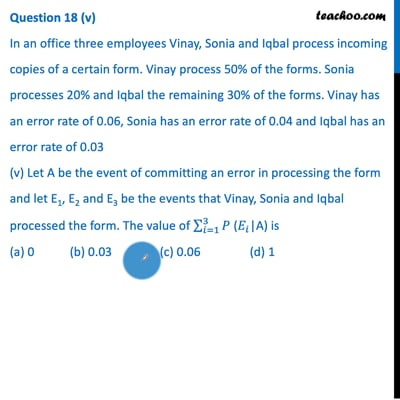This video is only available for Teachoo black users

Introducing your new favourite teacher - Teachoo Black, at only ₹83 per month

### Transcript

Question 18 In an office three employees Vinay, Sonia and Iqbal process incoming copies of a certain form. Vinay process 50% of the forms. Sonia processes 20% and Iqbal the remaining 30% of the forms. Vinay has an error rate of 0.06, Sonia has an error rate of 0.04 and Iqbal has an error rate of 0.03 Based on the above information answer the following: Question 18 (i) In an office three employees Vinay, Sonia and Iqbal process incoming copies of a certain form. Vinay process 50% of the forms. Sonia processes 20% and Iqbal the remaining 30% of the forms. Vinay has an error rate of 0.06, Sonia has an error rate of 0.04 and Iqbal has an error rate of 0.03 Based on the above information answer the following: (i) The conditional probability that an error is committed in processing given that Sonia processed the form is (a) 0.0210 (b) 0.04 (c) 0.47 (d) 0.06 Let A : Vinay processes the form B : Sonia Processes the form C : Iqbal processes the form We need to find The conditional probability that an error is committed in processing given that Sonia processed the form i.e. P(E|B) Since Sonia’s error rate 0.04 P(E|B) = 0.04 So, (b) is correct Question 18 (ii) In an office three employees Vinay, Sonia and Iqbal process incoming copies of a certain form. Vinay process 50% of the forms. Sonia processes 20% and Iqbal the remaining 30% of the forms. Vinay has an error rate of 0.06, Sonia has an error rate of 0.04 and Iqbal has an error rate of 0.03 (ii)The probability that Sonia processed the form and committed an error is : (a) 0.005 (b) 0.006 (c) 0.008 (d) 0.68 Probability that Sonia processed the form and committed an error = 20% × 0.04 = 20/100 × 0.04 = 0.008 So, (c) is correct Question 18 (iii) In an office three employees Vinay, Sonia and Iqbal process incoming copies of a certain form. Vinay process 50% of the forms. Sonia processes 20% and Iqbal the remaining 30% of the forms. Vinay has an error rate of 0.06, Sonia has an error rate of 0.04 and Iqbal has an error rate of 0.03 (iii)The total probability of committing an error in processing the form is (a) 0 (b) 0.047 (c) 0.234 (d) 1 The total probability of committing an error = Probability Vinay processes the form × Vinay’s error rate + Probability Sonia processes the form × Sonia’s error rate + Probability Iqbal processes the form × Iqbal’s error rate = 50% × 0.06 + 20% × 0.04 + 30% × 0.03 = 5/10 × 0.06 + 2/10 × 0.04 + 3/10 × 0.03 = 0.030 + 0.008 + 0.009 = 0.047 So, (b) is the correct answer Question 18 In an office three employees Vinay, Sonia and Iqbal process incoming copies of a certain form. Vinay process 50% of the forms. Sonia processes 20% and Iqbal the remaining 30% of the forms. Vinay has an error rate of 0.06, Sonia has an error rate of 0.04 and Iqbal has an error rate of 0.03 (iv) The manager of the company wants to do a quality check. During inspection he selects a form at random from the days output of processed forms. If the form selected at random has an error, the probability that the form is NOT processed by Vinay is : (a) 1 (b) 30/47 (c) 20/47 (d) 17/47 We first find The conditional probability that an error is committed in processing given that Vijay processed the form i.e. P(A|E) Question 18 In an office three employees Vinay, Sonia and Iqbal process incoming copies of a certain form. Vinay process 50% of the forms. Sonia processes 20% and Iqbal the remaining 30% of the forms. Vinay has an error rate of 0.06, Sonia has an error rate of 0.04 and Iqbal has an error rate of 0.03 (iv) The manager of the company wants to do a quality check. During inspection he selects a form at random from the days output of processed forms. If the form selected at random has an error, the probability that the form is NOT processed by Vinay is : (a) 1 (b) 30/47 (c) 20/47 (d) 17/47 We first find The Probability that an error is committed in processing given that Vijay processed the form i.e. P(A|E) Now, P(A|E) = (𝑷(𝑨). 𝑷(𝑬|𝑨))/(𝑷(𝑨). 𝑷(𝑬|𝑨) + 𝑷(𝑩). 𝑷(𝑬|𝑩) + 𝑷(𝑪). 𝑷(𝑬|𝑪)) = (5/10 × 0.06)/(5/10 × 0.06 + 2/10 × 0.04 + 3/10 × 0.03) = (5 × 0.06 )/(5 × 0.06 + 2 × 0.04 + 3 × 0.03) = (0.30 )/(0.30 + 0.08 + 0.09) = (0.30 )/0.47 = (𝟑𝟎 )/𝟒𝟕 Now, Probability form has an error but was NOT Processed by Vinay = 1 − P(A|E) = 1 − (30 )/47 = (𝟏𝟕 )/𝟒𝟕 So, (d) is the correct answer Question 18 (v) In an office three employees Vinay, Sonia and Iqbal process incoming copies of a certain form. Vinay process 50% of the forms. Sonia processes 20% and Iqbal the remaining 30% of the forms. Vinay has an error rate of 0.06, Sonia has an error rate of 0.04 and Iqbal has an error rate of 0.03 (v) Let A be the event of committing an error in processing the form and let E1, E2 and E3 be the events that Vinay, Sonia and Iqbal processed the form. The value of ∑_(𝑖=1)^3▒𝑃 (𝐸_𝑖|A) is (a) 0 (b) 0.03 (c) 0.06 (d) 1 Now, ∑_(𝑖=1)^3▒𝑃 (𝐸_𝑖|A) = P(E1|A) + P(E2|A) + P(E3|A) = (𝑃(𝐸_1 ). 𝑃(𝐴|𝐸_1))/(𝑃(𝐸_1 ). 𝑃(𝐴|𝐸_1) + 𝑃(𝐸_2 ). 𝑃(𝐴|𝐸_2) + 𝑃(𝐸_3 ). 𝑃(𝐴|𝐸_1)) + (𝑃(𝐸_2 ). 𝑃(𝐴|𝐸_2))/(𝑃(𝐸_1 ). 𝑃(𝐴|𝐸_1) + 𝑃(𝐸_2 ). 𝑃(𝐴|𝐸_2) + 𝑃(𝐸_3 ). 𝑃(𝐴|𝐸_1)) + (𝑃(𝐸_3 ). 𝑃(𝐴|𝐸_3))/(𝑃(𝐸_1 ). 𝑃(𝐴|𝐸_1) + 𝑃(𝐸_2 ). 𝑃(𝐴|𝐸_2) + 𝑃(𝐸_3 ). 𝑃(𝐴|𝐸_1)) = (𝑃(𝐸_1 ). 𝑃(𝐴|𝐸_1) + 𝑃(𝐸_2 ). 𝑃(𝐴|𝐸_2) + 𝑃(𝐸_3 ). 𝑃(𝐴|𝐸_1))/(𝑃(𝐸_1 ). 𝑃(𝐴|𝐸_1) + 𝑃(𝐸_2 ). 𝑃(𝐴|𝐸_2) + 𝑃(𝐸_3 ). 𝑃(𝐴|𝐸_1)) = 1 So, (d) is the correct answer